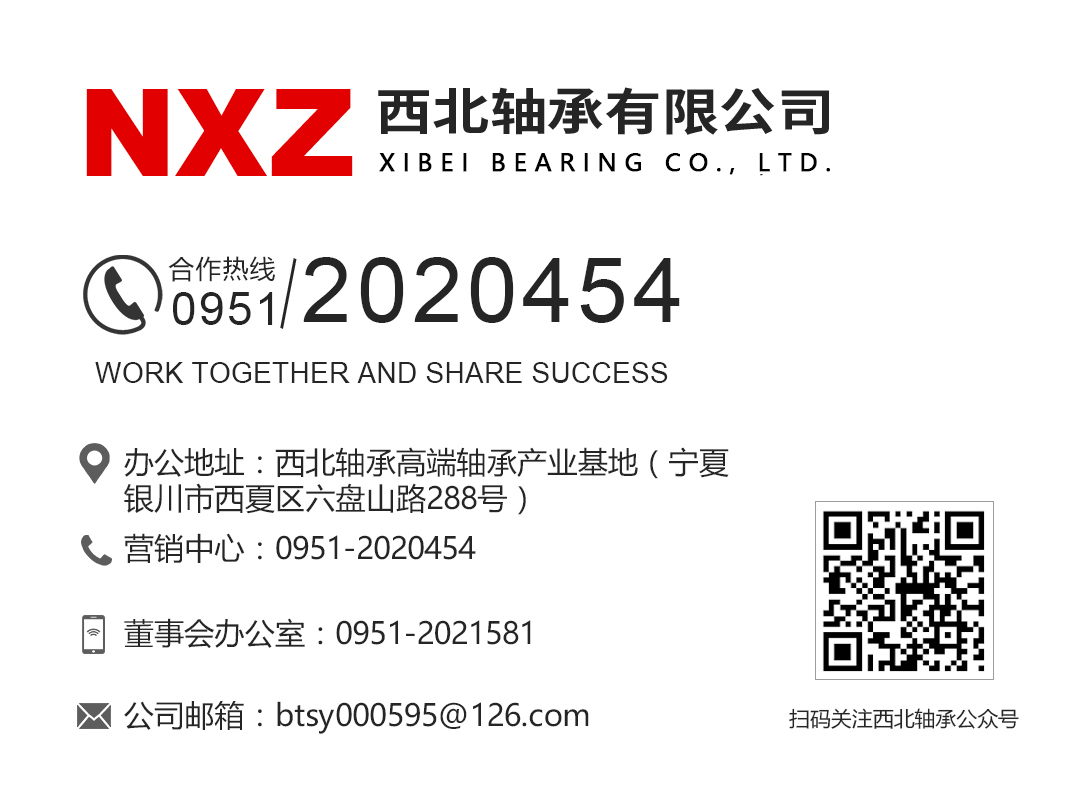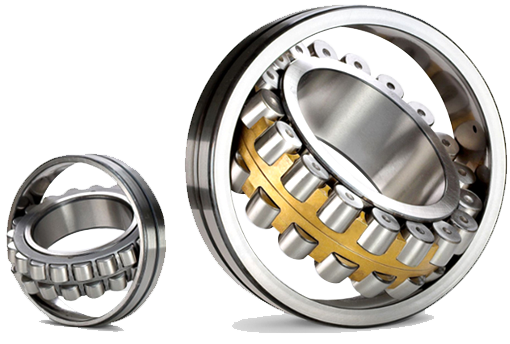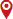首页> News > Information

### [knowledge] are the concepts of strength and stiffness always confused? I'll understand after reading this article

Many people are always confused about the concepts of strength and stiffness in mechanics. Let's talk about our understanding today. The book says that in order to ensure the normal operation of the mechanical system or the whole structure, each part or component must be able to work normally. The task of engineering component safety design is to ensure that the component has sufficient strength, stiffness and stability.

Stability is well understood, the ability to maintain or restore the original equilibrium form under force. For example, the thin rod under pressure suddenly bends, the load-bearing of thin-walled members folds, or the instability of building columns leads to collapse, which is well understood. Today, I will mainly talk about the understanding of stiffness and strength.

strength

one

Definition: the ability of components or parts to resist damage (fracture) or significant deformation under the action of external force.

For example, Sun Yue regarded the iPad as a scale. When he stood up, the iPad screen cracked, which is not strong enough. For example, when Wuhan looks at the sea every summer, many big branches are broken by the wind, which is not strong enough.

Strength is a parameter that reflects the failure of materials such as fracture. Generally, strength includes tensile strength and compressive strength, which is the amount of material failure when the stress reaches. The unit of strength is generally MPa.

one point one

Failure type

Brittle fracture: sudden fracture without obvious plastic deformation. For example, the fracture of cast iron specimen along the cross section during tension and the fracture of cast iron specimen with circular section along the oblique section during torsion.

Plastic yield: the material produces significant plastic deformation and makes the component lose its working capacity. For example, the low-carbon steel sample will have significant plastic deformation during tension or torsion.

one point two

strength theory

Maximum tensile stress theory: as long as the maximum tensile stress at one point in the member σ 1. The ultimate stress under unidirectional stress state is reached σ b. Brittle fracture will occur in materials. Therefore, the conditions for brittle fracture failure of components with dangerous points in complex stress state are as follows: σ 1= σ b。 Therefore, the strength conditions established according to the first strength theory are: σ 1≤[ σ] 。

Maximum tensile strain theory: as long as the maximum tensile strain ε 1 reach the limit value under unidirectional stress state ε u. The material will undergo brittle fracture failure, ε 1= ε u。 From the generalized Hooke's Law: ε 1=[ σ 1-u( σ 2+ σ 3) ] / E, so σ 1-u( σ 2+ σ 3)= σ b。 The strength conditions established according to the second strength theory are: σ 1-u( σ 2+ σ 3)≤[ σ]。

Maximum shear stress theory: as long as the maximum shear stress τ Max reaches the ultimate shear stress under unidirectional stress state τ 0, the material will yield failure. τ max= τ 0 According to the stress formula on the inclined section of axial tension τ 0= σ s/2( σ S is the normal stress on the cross section) obtained from the formula: τ max=( σ 1- σ 3)/2。 So the damage condition is rewritten as σ 1- σ 3= σ s。 The strength condition according to the third strength theory is: σ 1- σ 3≤[ σ]。

Shape change specific energy theory: as long as the shape change specific energy at a point in the component reaches the limit value under unidirectional stress state, the material will yield failure. Therefore, according to the fourth strength theory, the strength condition is: sqrt（ σ one ²+σ two ²+σ three ²-σ one σ 2- σ two σ 3- σ three σ 1)<[ σ]

rigidity

two

Definition: refers to the ability of members or parts to resist elastic deformation or displacement under the action of external force, that is, the elastic deformation or displacement shall not exceed the allowable range of the project.

Stiffness is a parameter reflecting the relationship between structural deformation and force, that is, the amount of deformation generated by how much force the structure is subjected to. In short, it is a spring, and the stiffness of the spring is the tensile force divided by the elongation. The unit of stiffness is generally n / m.

two point one

Stiffness type

When the applied load is a constant load, it is called static stiffness; When it is an alternating load, it is called dynamic stiffness. Static stiffness mainly includes structural stiffness and contact stiffness. Structural stiffness refers to the stiffness of the member itself, mainly including bending stiffness and torsional stiffness.

The bending stiffness is calculated according to the following formula: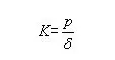Where, P is the static load (n), δ  Is the elastic deformation in the load direction（ μ m)。 The torsional stiffness is calculated according to the following formula: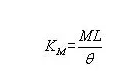Where, M is the applied torque (n · m), and l is the distance from the torque to the fixed end (m), θ  Is the torsion angle (°).

Connection between the two

three

Through the above theoretical understanding of strength and stiffness, compared with stiffness, the definition of strength is aimed at the failure under the action of external force; The failure types are classified as plastic yield and brittle fracture, which is associated with the stress-strain curve during tension. As shown in the figure.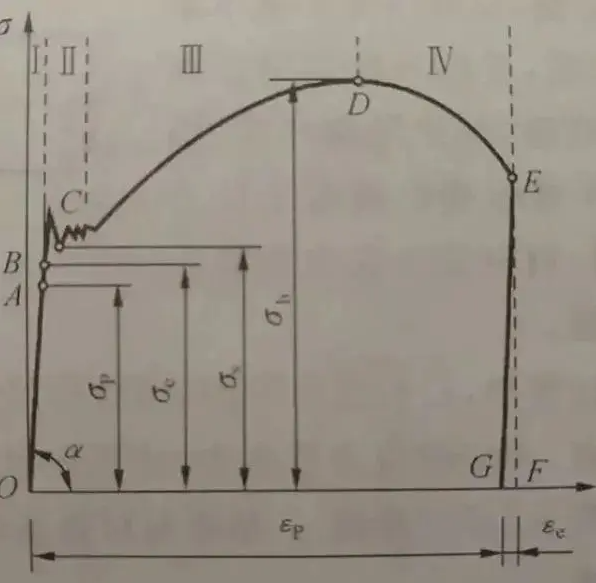The curve in the figure can be divided into four stages: I. elastic deformation stage;

2. Yield stage;

3. Strengthening stage;

4. Local necking stage.

The definition of stiffness is to resist elastic deformation, which is carried out in the first stage. Hooke's law is satisfied under elastic action. Observe the calculation formula of bending stiffness and torsional stiffness under static load, which is similar to Hooke's law. It can be inferred that the measurement of stiffness is only carried out in the elastic deformation stage.

After entering the next stage, the plastic strain or residual strain will not disappear in the tensile process. Under the stress-strain curve, the stress is almost unchanged, but the strain increases significantly. At this time, the stress is the yield limit, and the material enters the failure stage of plastic yield. After entering the strengthening stage, the strain increases with the increase of stress, and finally reaches the strength limit. It can be seen that the strength is measured before the elastic limit.

To sum up, it can be concluded that both stiffness and strength are measured in the failure stage of parts, while stiffness can be measured by stress and strength can be measured by deformation. In the strain process, the stiffness is in the previous stage and the strength is in the later stage. Therefore, in the condition measurement of part failure, as long as the stiffness requirements are met, enough stress can be resisted in the elastic deformation stage, and the strength meets the requirements of the part on this premise. According to this relationship, there will be various designs in actual production, such as the shaft in mechanical equipment. Usually, the size of the shaft is determined according to the strength conditions, and then the stiffness is checked according to the stiffness conditions. Therefore, the stiffness requirements of precision machinery for the shaft are set very high, and the design of its section size is often controlled by the stiffness conditions.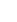办公地址：西北轴承高端轴承产业基地（银川市西夏区六盘山路388号） 邮政编码：750021 营销中心：0951-2020454 董事会办公室：0951-8697187 公司邮箱：nxzgroup@nxz.com.cn